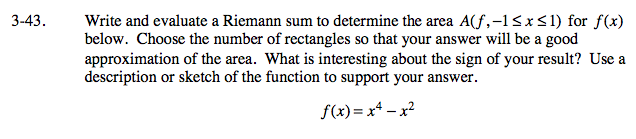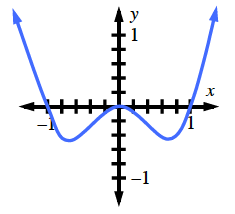### Home > CALC > Chapter Ch3 > Lesson 3.2.1 > Problem3-43

3-43.

Write and evaluate a Riemann sum to determine the area A(f, −1 ≤ x ≤ 1) for f(x) below. Choose the number of rectangles so that your answer will be a good approximation of the area. What is interesting about the sign of your result? Use a description or sketch of the function to support your answer. Homework Help ✎
f(x) = x4 − x2Your answer should be relatively close to ≈ −0.26667. The answer is negative because the function is below the x-axis on this interval.